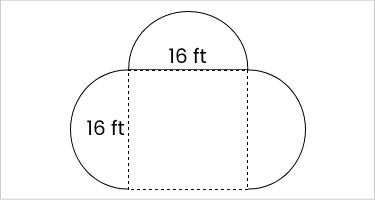### Still have math questions?

Geometry
Question

Find the perimeter of the composite geometric figure, which consists of three semicircles and a square. Use $$\pi\approx3.14$$.(Round to the nearest hundredth.)$$91.36\text{ ft}^2$$